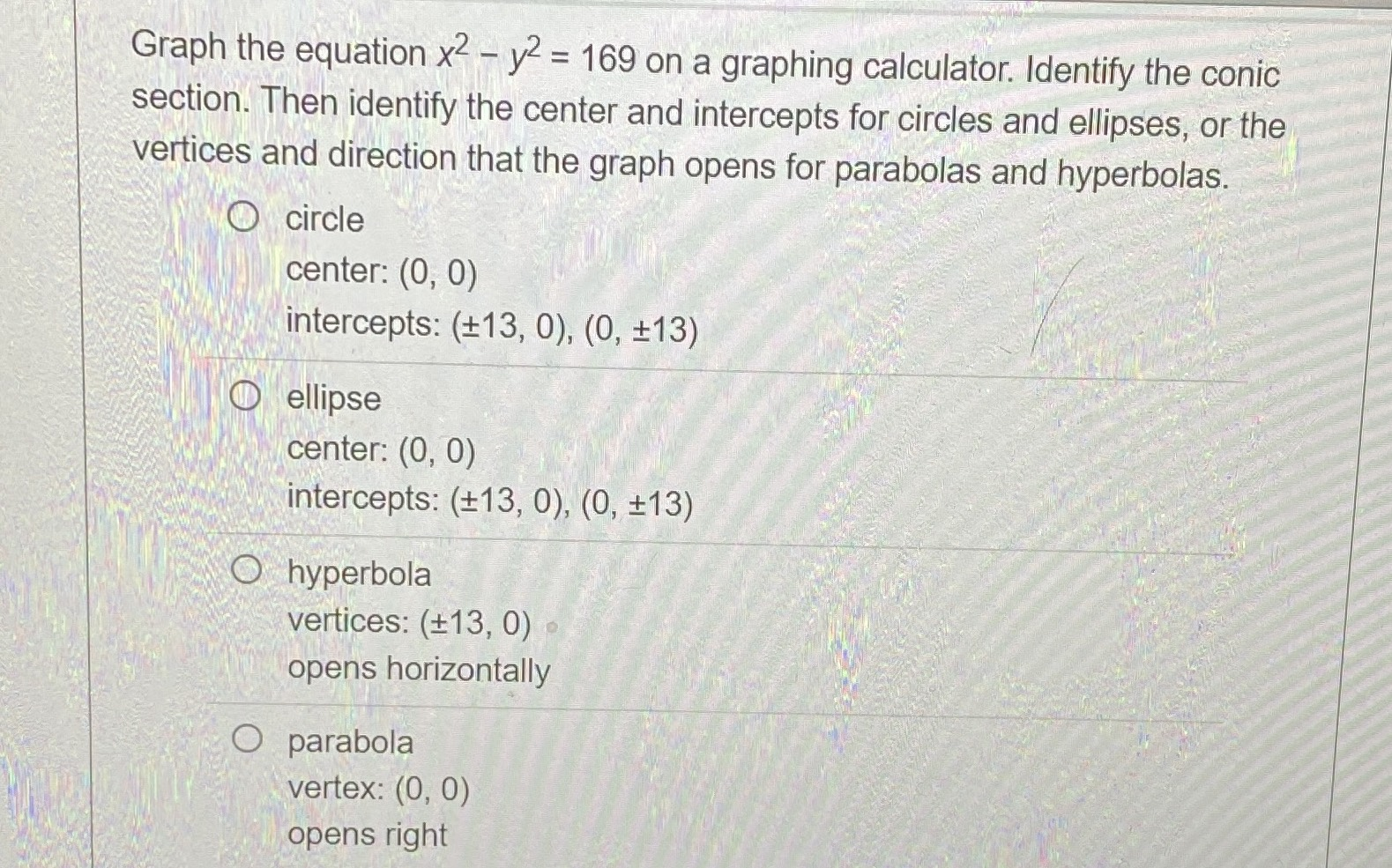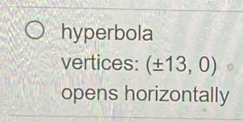### Still have math questions?

Algebra
QuestionGraph the equation $$x ^ { 2 } - y ^ { 2 } = 169$$ on a graphing calculator. Identify the conic section. Then identify the center and intercepts for circles and ellipses, or the vertices and direction that the graph opens for parabolas and hyperbolas.

circle

center: $$( 0,0 )$$

intercepts: $$( \pm 13,0 ) , ( 0 , \pm 13 )$$

ellipse

center: $$( 0,0 )$$

intercepts: $$( \pm 13,0 ) , ( 0 , \pm 13 )$$

hyperbola

vertices: $$( \pm 13,0 )$$

opens horizontally

parabola

center: $$( 0,0 )$$ vertex: $$( 0,0 )$$

opens right